# Conditional Control Flow Constructs Sequential Control Flow Execution

• Slides: 29Conditional Control Flow Constructs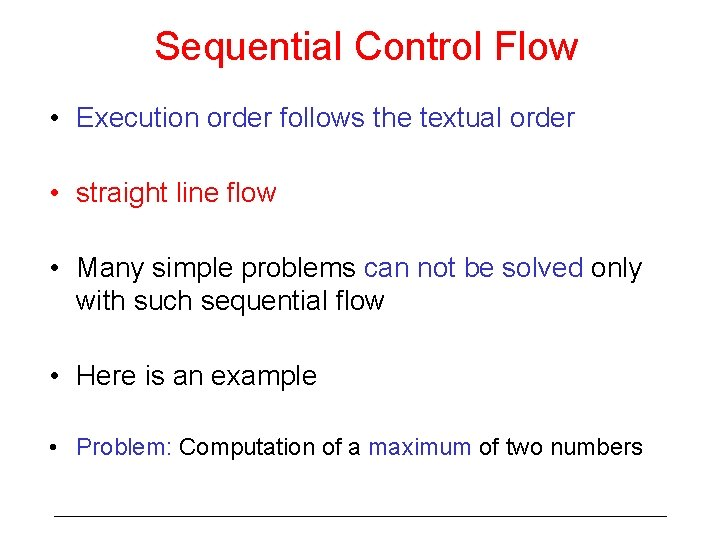Sequential Control Flow • Execution order follows the textual order • straight line flow • Many simple problems can not be solved only with such sequential flow • Here is an example • Problem: Computation of a maximum of two numbers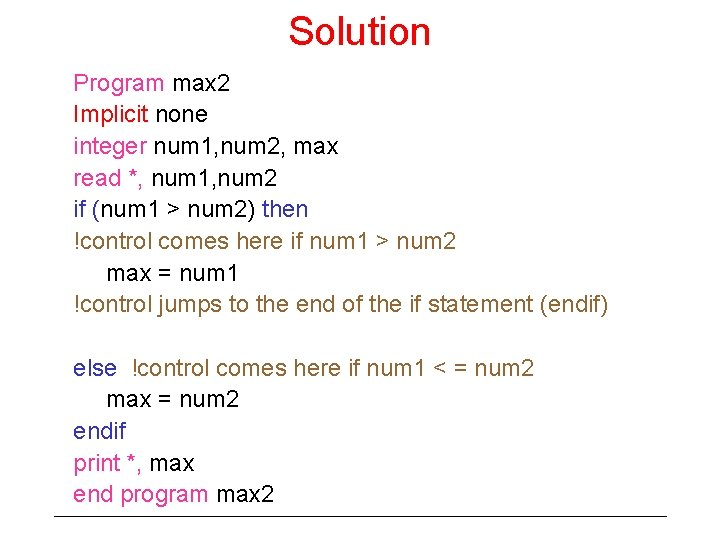Solution Program max 2 Implicit none integer num 1, num 2, max read *, num 1, num 2 if (num 1 > num 2) then !control comes here if num 1 > num 2 max = num 1 !control jumps to the end of the if statement (endif) else !control comes here if num 1 < = num 2 max = num 2 endif print *, max end program max 2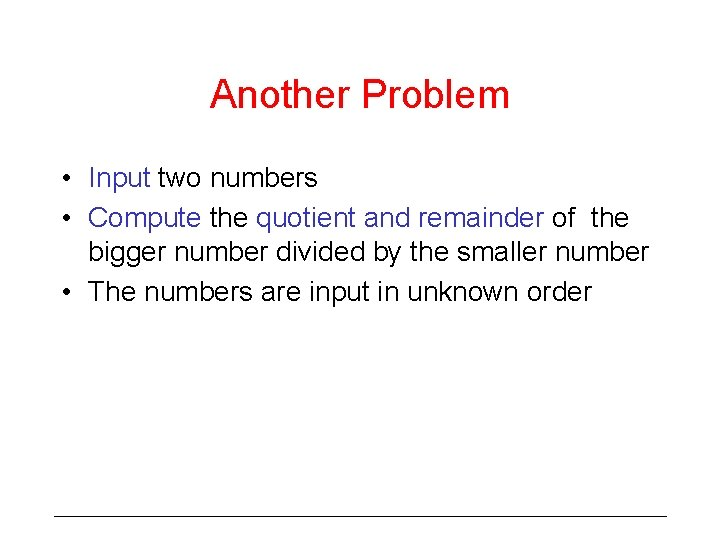Another Problem • Input two numbers • Compute the quotient and remainder of the bigger number divided by the smaller number • The numbers are input in unknown orderSolution Program Quo_Rem 1 Implicit none integer num 1, num 2, quotient, remainder read *, num 1, num 2 if (num 1 > num 2) then quotient = num 1/num 2 ! / is integer division remainder = num 1 – (quotient * num 2) else quotient = num 2/num 1 remainder = num 2 – (quotient * num 1) endif print *, quotient, remainder end program Quo_Rem 1Problems with the solution What happens if num 1 or num 2 is negative? – Problem Specification needs to be clarified What if one of the numbers is 0? – Divide by zero leads to overflow – Should be avoided – need to test it before dividing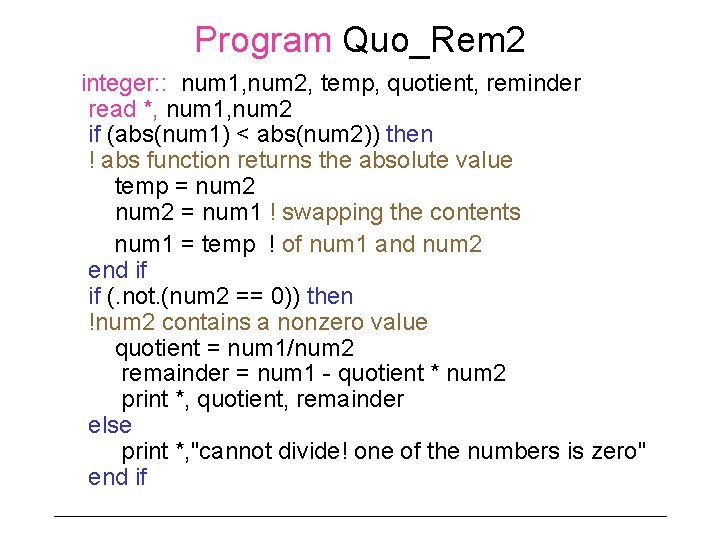Program Quo_Rem 2 integer: : num 1, num 2, temp, quotient, reminder read *, num 1, num 2 if (abs(num 1) < abs(num 2)) then ! abs function returns the absolute value temp = num 2 = num 1 ! swapping the contents num 1 = temp ! of num 1 and num 2 end if if (. not. (num 2 == 0)) then !num 2 contains a nonzero value quotient = num 1/num 2 remainder = num 1 - quotient * num 2 print *, quotient, remainder else print *, "cannot divide! one of the numbers is zero" end if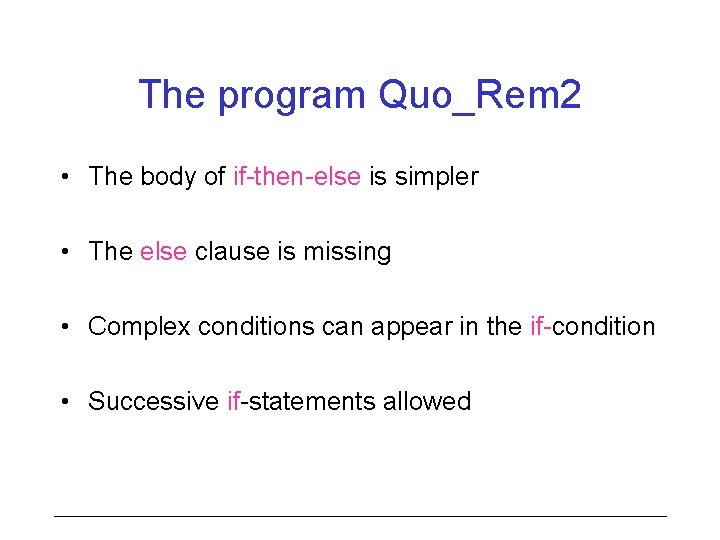The program Quo_Rem 2 • The body of if-then-else is simpler • The else clause is missing • Complex conditions can appear in the if-condition • Successive if-statements allowed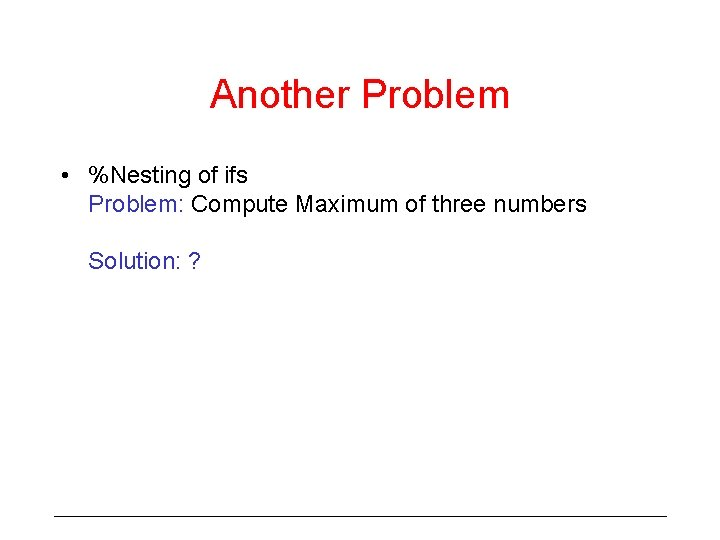Another Problem • %Nesting of ifs Problem: Compute Maximum of three numbers Solution: ?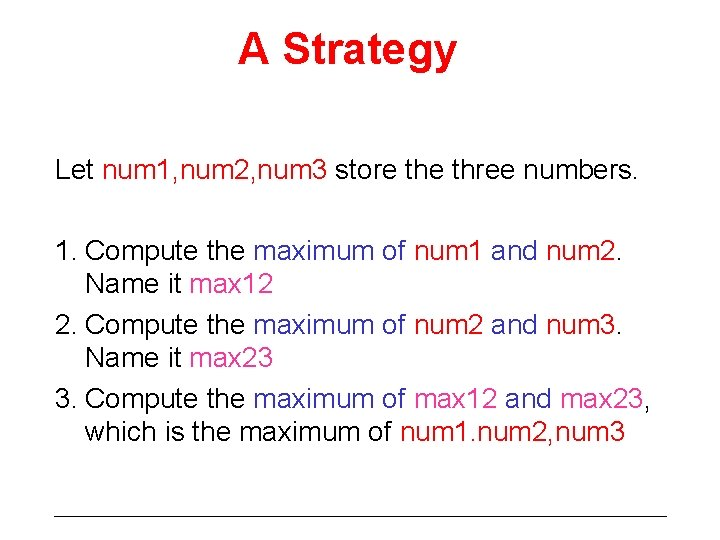A Strategy Let num 1, num 2, num 3 store three numbers. 1. Compute the maximum of num 1 and num 2. Name it max 12 2. Compute the maximum of num 2 and num 3. Name it max 23 3. Compute the maximum of max 12 and max 23, which is the maximum of num 1. num 2, num 3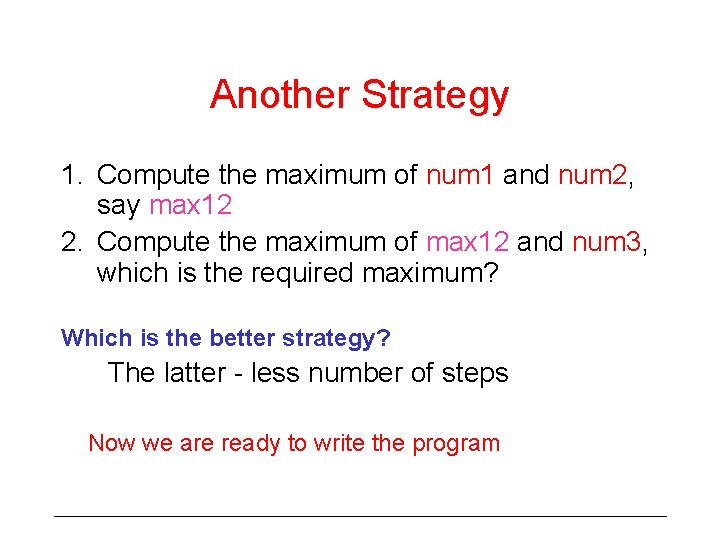Another Strategy 1. Compute the maximum of num 1 and num 2, say max 12 2. Compute the maximum of max 12 and num 3, which is the required maximum? Which is the better strategy? The latter - less number of steps Now we are ready to write the program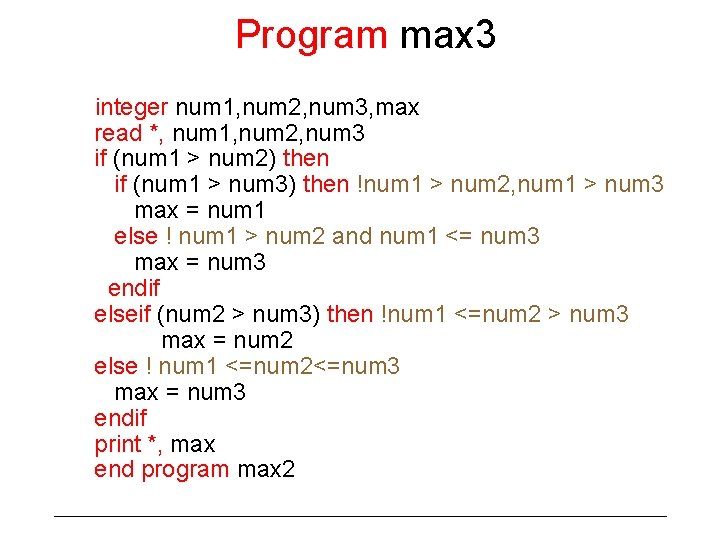Program max 3 integer num 1, num 2, num 3, max read *, num 1, num 2, num 3 if (num 1 > num 2) then if (num 1 > num 3) then !num 1 > num 2, num 1 > num 3 max = num 1 else ! num 1 > num 2 and num 1 <= num 3 max = num 3 endif elseif (num 2 > num 3) then !num 1 <=num 2 > num 3 max = num 2 else ! num 1 <=num 2<=num 3 max = num 3 endif print *, max end program max 2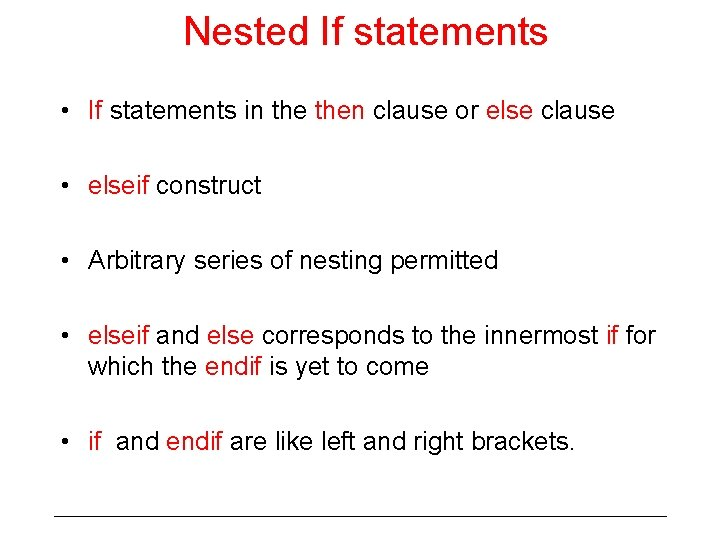Nested If statements • If statements in then clause or else clause • elseif construct • Arbitrary series of nesting permitted • elseif and else corresponds to the innermost if for which the endif is yet to come • if and endif are like left and right brackets.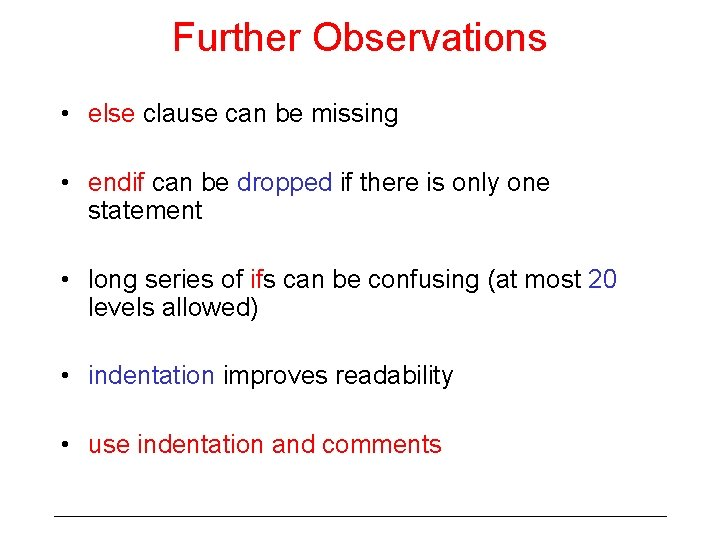Further Observations • else clause can be missing • endif can be dropped if there is only one statement • long series of ifs can be confusing (at most 20 levels allowed) • indentation improves readability • use indentation and commentsIf conditions • Control flow branches in conditional statements • Branching decided by evaluating the conditions • conditions are expressions of a new type called LOGICAL • Examples: (x > 0), (z == 1), . NOT. (num == 2) • All these involve relational operators: >, == • Relational operators are defined over many data types to compare values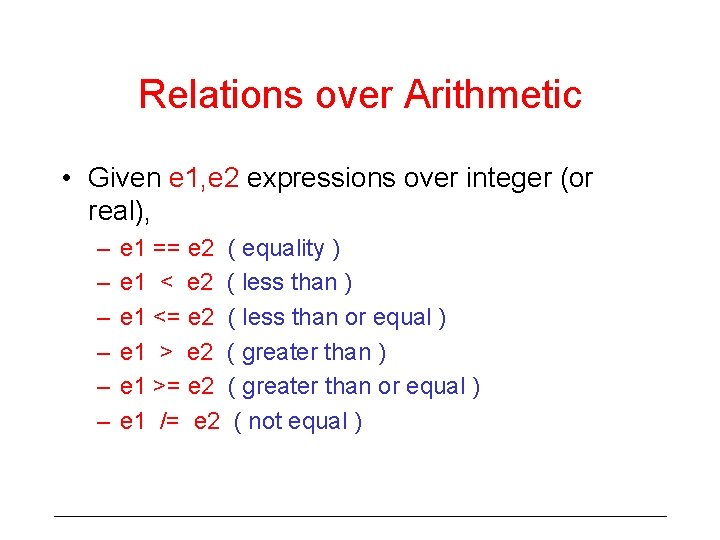Relations over Arithmetic • Given e 1, e 2 expressions over integer (or real), – – – e 1 == e 2 ( equality ) e 1 < e 2 ( less than ) e 1 <= e 2 ( less than or equal ) e 1 > e 2 ( greater than ) e 1 >= e 2 ( greater than or equal ) e 1 /= e 2 ( not equal )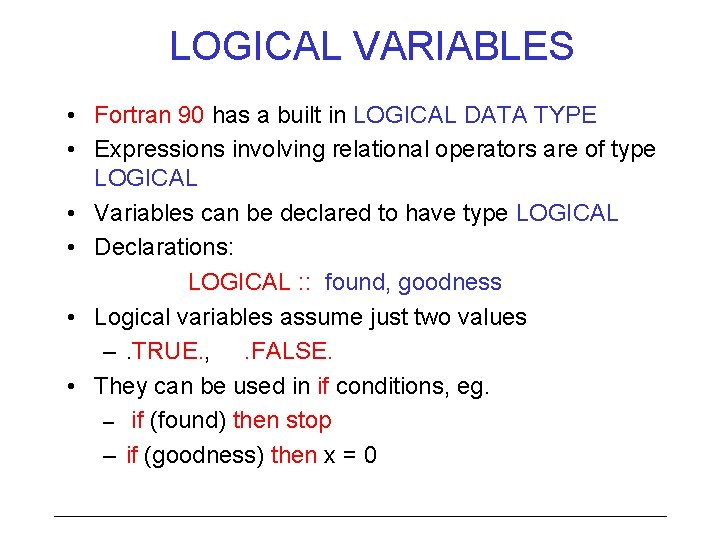LOGICAL VARIABLES • Fortran 90 has a built in LOGICAL DATA TYPE • Expressions involving relational operators are of type LOGICAL • Variables can be declared to have type LOGICAL • Declarations: LOGICAL : : found, goodness • Logical variables assume just two values –. TRUE. , . FALSE. • They can be used in if conditions, eg. – if (found) then stop – if (goodness) then x = 0LOGICAL OPERATORS • Operators over logical type values • They are. not. , . and. , . or. , . eqv. , . neqv. • . not. p is. true. • iff p is. false. p. and. q is. true. iff both p and q are. false. • p. or. q is. true. iff one of (or both) p, q. true. • p. eqv. q is. true. iff both p and q has the same truth value • p. neqv. q is. true. iff both p and q have different truth valuesOperator precedence • A general logical expression may include arithmetic, relational and logical operators • operator precedence defined to specify order of evaluation • arithmetic operators are evaluated first followed by relational operators • logical operators are evaluated last • precedence amongst logical operators. not. , . and. , . or. , . eqv. and. neqv.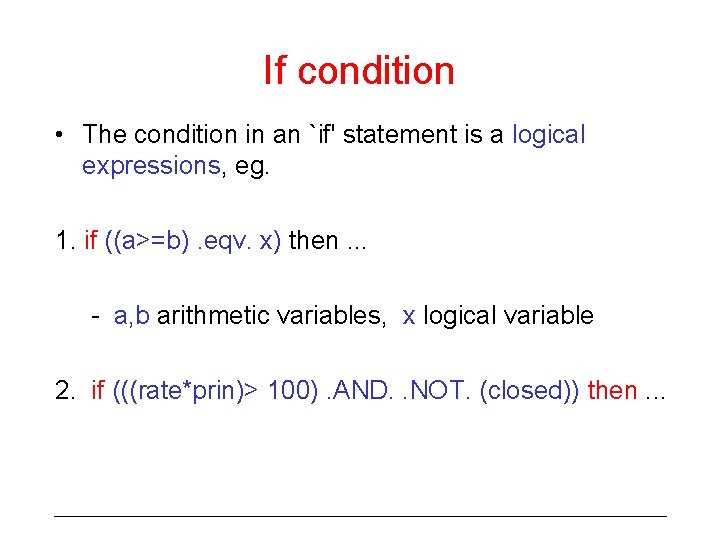If condition • The condition in an `if' statement is a logical expressions, eg. 1. if ((a>=b). eqv. x) then. . . - a, b arithmetic variables, x logical variable 2. if (((rate*prin)> 100). AND. . NOT. (closed)) then. . .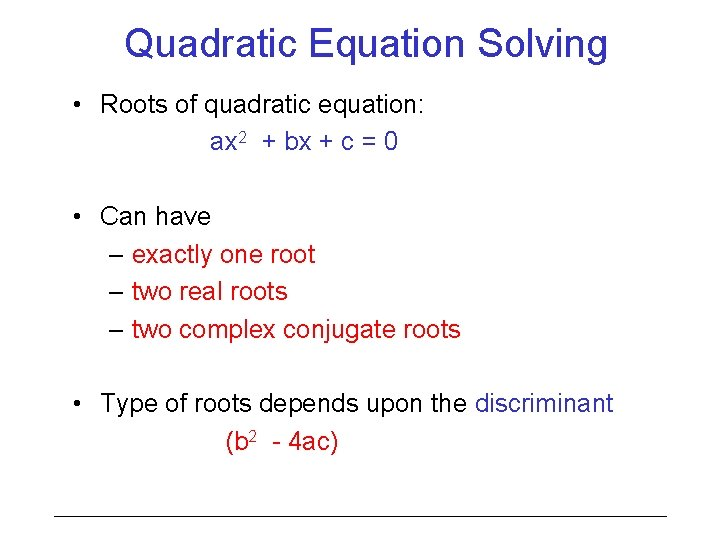Quadratic Equation Solving • Roots of quadratic equation: ax 2 + bx + c = 0 • Can have – exactly one root – two real roots – two complex conjugate roots • Type of roots depends upon the discriminant (b 2 - 4 ac)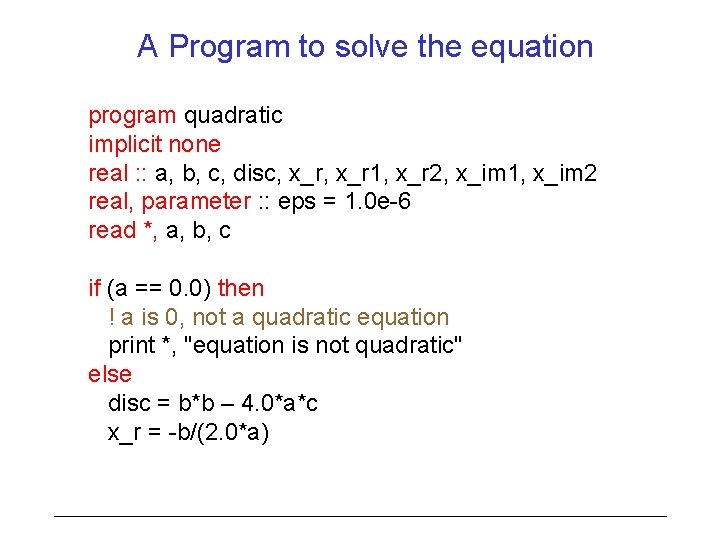A Program to solve the equation program quadratic implicit none real : : a, b, c, disc, x_r 1, x_r 2, x_im 1, x_im 2 real, parameter : : eps = 1. 0 e-6 read *, a, b, c if (a == 0. 0) then ! a is 0, not a quadratic equation print *, "equation is not quadratic" else disc = b*b – 4. 0*a*c x_r = -b/(2. 0*a)if ( abs(disc) < eps ) then ! discriminant nearly zero print *, "double real root", x_r elseif ( disc > 0 ) then ! two distinct real roots disc = sqrt(disc)/(2. 0*a) x_r 1 = x_r + disc ! disc temporary variable x_r 2 = x_r - disc print *, “two real roots”, x_r 1, “ and”, x_r 2 else ! disc is negative, complex conjugate roots x_im 1 = sqrt(-disc)/(2. 0*a) x_im 2 = - x_im 1 print *, "complex conjugate roots", x_r, "+", & x_im 1, "i and", x_r, "-", x_im 2, "i" endif end program quadratic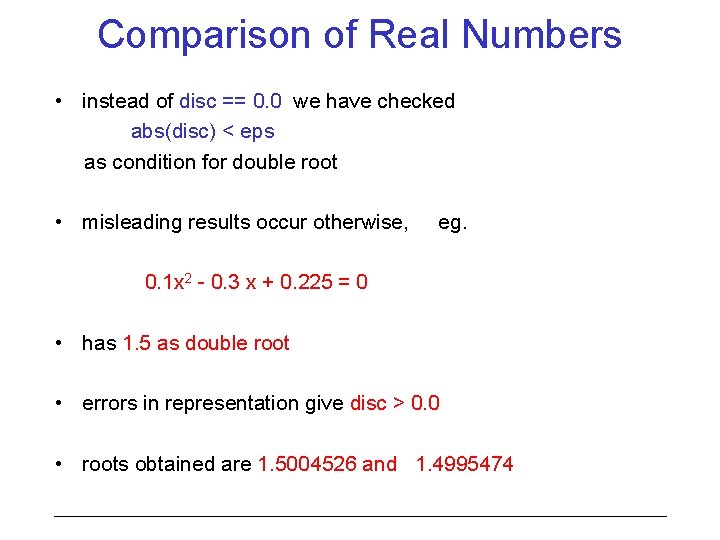Comparison of Real Numbers • instead of disc == 0. 0 we have checked abs(disc) < eps as condition for double root • misleading results occur otherwise, eg. 0. 1 x 2 - 0. 3 x + 0. 225 = 0 • has 1. 5 as double root • errors in representation give disc > 0. 0 • roots obtained are 1. 5004526 and 1. 4995474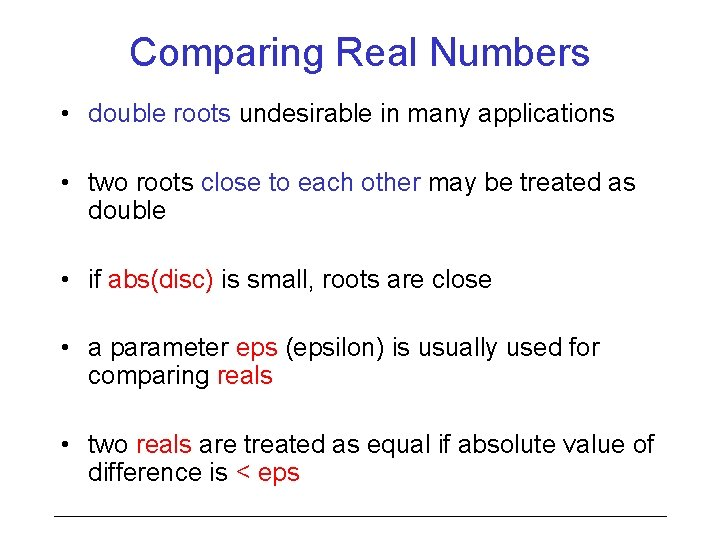Comparing Real Numbers • double roots undesirable in many applications • two roots close to each other may be treated as double • if abs(disc) is small, roots are close • a parameter eps (epsilon) is usually used for comparing reals • two reals are treated as equal if absolute value of difference is < eps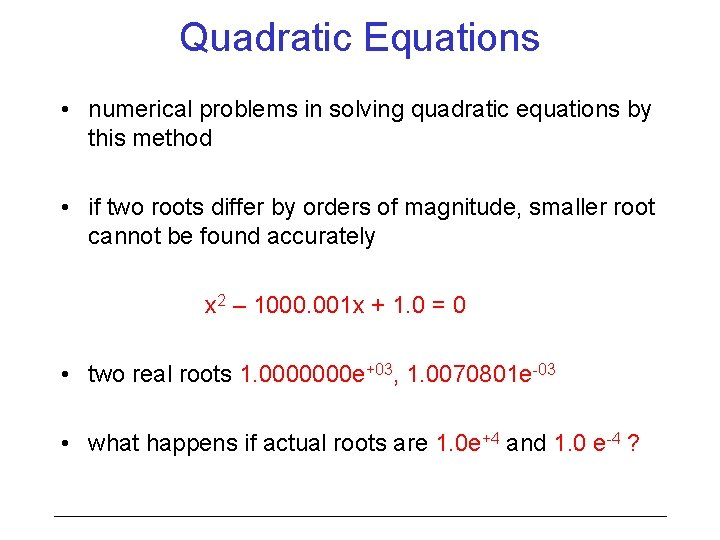Quadratic Equations • numerical problems in solving quadratic equations by this method • if two roots differ by orders of magnitude, smaller root cannot be found accurately x 2 – 1000. 001 x + 1. 0 = 0 • two real roots 1. 0000000 e+03, 1. 0070801 e-03 • what happens if actual roots are 1. 0 e+4 and 1. 0 e-4 ?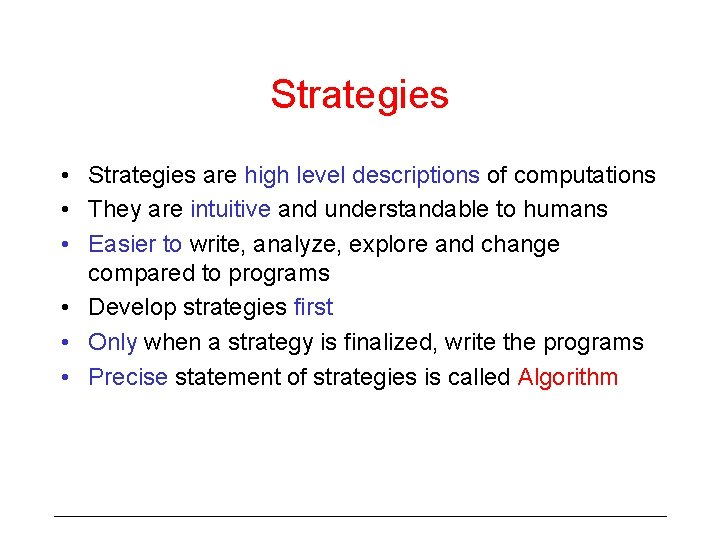Strategies • Strategies are high level descriptions of computations • They are intuitive and understandable to humans • Easier to write, analyze, explore and change compared to programs • Develop strategies first • Only when a strategy is finalized, write the programs • Precise statement of strategies is called Algorithm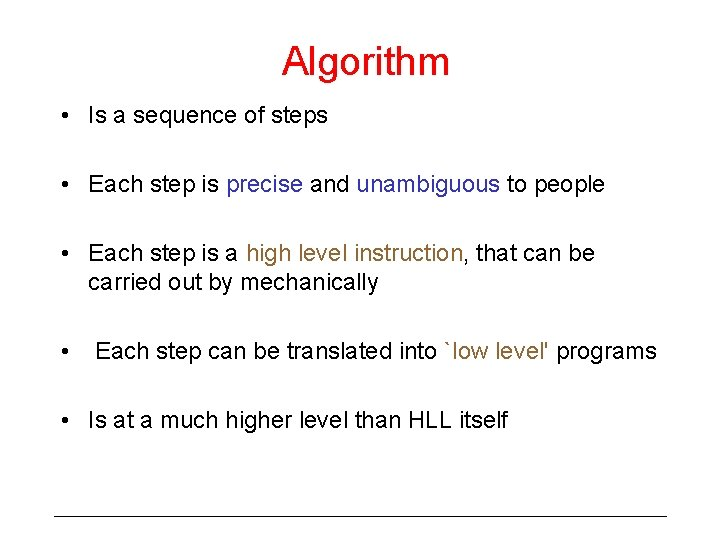Algorithm • Is a sequence of steps • Each step is precise and unambiguous to people • Each step is a high level instruction, that can be carried out by mechanically • Each step can be translated into `low level' programs • Is at a much higher level than HLL itselfAnalyzing algorithms • Before writing the program, analyze and choose the efficient algorithm • Metrics for algorithm – Number of steps – Complexity of steps • number of primitive operations (like addition, multiplication, comparison)